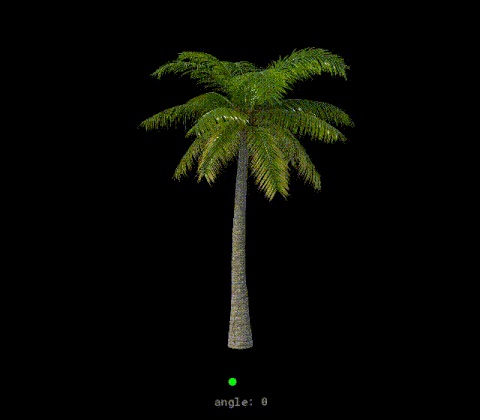# GMLscripts.com

Discuss and collaborate on GML scriptsYou are not logged in.

## #1 2021-09-09 15:36:37

maras
MemberRegistered: 2021-04-25
Posts: 27
Website

### Draw a bent sprite

update 2. 12. 2022
https://github.com/mh-cz/draw_sprite_bent

Small performance boost and added return_pts to return the final transformed coordinates of each segment so you can maybe attach something to it

Expand/// draw_sprite_bent(spr, img_index, x, y, around_x, around_y, bend_angle, segments, xscale = 1, yscale = 1, rot = 0, color = c_white, alpha = 1, return_pts = -1)
//
// returns array of points if return_pts != -1
//
//	spr	sprite index, real
//	img_index	image index, real
//	x	draw it here, real
//	y	draw it here, real
//	around_x	bend the sprite around this x position, real
//	around_y	bend the sprite around this y position, real
//	bend_angle	apply angle, real
//	segments	the more segments, the better quality (but lower performance), real
//	xscale	scale on the x axis, real
//	yscale	scale on the y axis, real
//	rot	rotation of the final drawn texture (does not affect actual bending and around_x/y position), real
//	color	colour
//	alpha	alpha, real
//	return_pts	return array of transformed coords from bottom to top, real 0 to 1 (-1 is off)
//
//  THE IMAGE REQUIRES TO HAVE "Separate texture page" TICKED!
//
//  return_pts = 0 -> return points on the left side
//  return_pts = 0.5 -> return points in the middle
//  return_pts = 1 -> return points on the right side
//

global.BEND_SPR_DATA = [];

vertex_format_begin();
global.BEND_SPR_DATA = vertex_format_end(); // vf
global.BEND_SPR_DATA = vertex_create_buffer(); // b

function draw_sprite_bent(spr, img_index, x, y, around_x, around_y, bend_angle, segments, xscale = 1, yscale = 1, rot = 0, color = c_white, alpha = 1, return_pts = -1) {

var spr_w = sprite_get_width(spr);
var spr_h = sprite_get_height(spr);
var xoff = sprite_get_xoffset(spr) * xscale;
var yoff = sprite_get_yoffset(spr) * yscale;

segments = max(4, ceil(segments));
var step = spr_h / segments;
var angle_step = bend_angle / segments;

// split the sprite into triangles with UVs

var uv = array_create(2 + ceil((spr_h + 0.1) / step));
var ci = 0;

uv[ci++] = [1, 0];

var right = false;
for(var h = 0; h < spr_h + 0.1; h += step) {
uv[ci++] = [real(right), h/spr_h];
right = !right;
}
uv[ci++] = [real(right), 1];

// rotate the triangles around pivot and save the new coordinates

var counter = 0;
var angl = 0;
var uvlen = array_length(uv);
var coords = array_create(uvlen);
ci = 0;

var u, v, pivot_x, pivot_y, pdr, pds, xx, yy;

for(var i = uvlen-1; i > -1; i--) {

u = uv[i];
v = uv[i];

pivot_x = (around_x - (x - xoff)) / xscale;
pivot_y = (around_y - (y - yoff)) / yscale;

pdr = point_direction(pivot_x, pivot_y, u * spr_w, v * spr_h);
pds = point_distance(pivot_x, pivot_y, u * spr_w, v * spr_h);
xx = pivot_x + lengthdir_x(pds, pdr + angl);
yy = pivot_y + lengthdir_y(pds, pdr + angl);

coords[ci++] = [ xx * xscale, yy * yscale, (counter == 1), u, v ];

if counter++ == 2 {
counter = 0;
if i == 0 break;
i += 2;
angl += angle_step;
}
}

var vf = global.BEND_SPR_DATA;
var b = global.BEND_SPR_DATA;

vertex_begin(b, vf);

var final_pts = return_pts != -1 ? array_create(floor(segments/2)) : [];
var ptsi = 0;
var prev_px = undefined;
var prev_py = undefined;
var this_px = 0;
var this_py = 0;

var c_prev, c_next, c_curr;

for(var i = 0; i < ci; i++) {

// connect corners so the image can stretch

c_curr = coords[i];

if i == clamp(i, 2, ci-3) {

c_prev = coords[i-2];
c_next = coords[i+2];

if c_prev {
c_curr = c_prev;
c_curr = c_prev;
}
if c_next {
c_curr = c_next;
c_curr = c_next;
}
}

// apply rotation and draw

xx = c_curr - xoff;
yy = c_curr - yoff;

if rot != 0 {
pdr = point_direction(0, 0, xx, yy);
pds = point_distance(0, 0, xx, yy);
xx = lengthdir_x(pds, pdr + rot);
yy = lengthdir_y(pds, pdr + rot);
}

vertex_position(b, x + xx, y + yy);
vertex_colour(b, color, alpha);
vertex_texcoord(b, c_curr, c_curr);

if return_pts != -1 and i > 0 and i % 6 == 1 {

prev_px = x + coords[i-1] - xoff;
prev_py = y + coords[i-1] - yoff;
this_px = x + xx;
this_py = y + yy;

var ds = point_distance(this_px, this_py, prev_px, prev_py) * return_pts;
var dr = point_direction(this_px, this_py, prev_px, prev_py);

final_pts[ptsi++] = [ this_px + lengthdir_x(ds, dr), this_py + lengthdir_y(ds, dr) ];
}
}

vertex_end(b);
vertex_submit(b, pr_trianglelist, sprite_get_texture(spr, img_index));

return final_pts;
}

Make sure to tick "Separate texture page" otherwise it will be glitched

The green dot is around_x and around_y attached to the mouse cursor
Adjusting the bend_angle with mouse wheel
40 segmentsLast edited by maras (2022-12-02 06:51:33)

I'm on the official GM discord > maras#5104

Offline

## #2 2022-06-27 04:42:12

gnysek
MemberRegistered: 2011-12-29
Posts: 33

### Re: Draw a bent sprite

This is genius! I wanted to buy Spine for \$330 to apply similar effect in my game for trees, and here I've got same thing for free (and probably faster, as it's doesn't need to process thousands of additional variables).

Offline

## #3 2023-01-11 08:05:29

Monvario
Member
From: France
Registered: 2023-01-11
Posts: 1
Website

### Re: Draw a bent sprite

I'm not quite sure, but I'll need to check another way to soolve this problem.

Offline